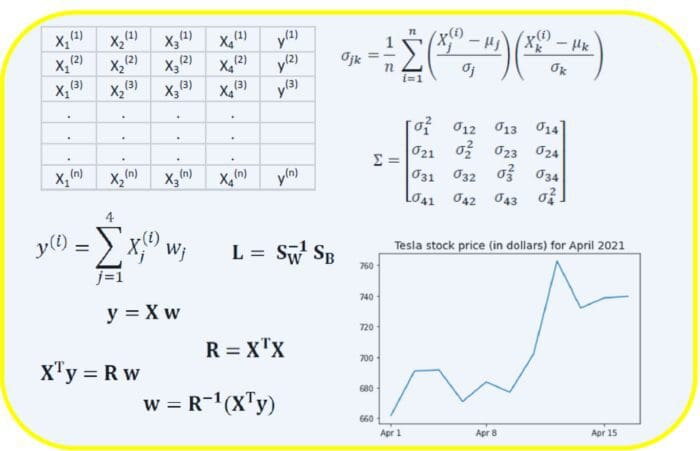# Linear Algebra for Data Science

In this article, we discuss the importance of linear algebra in data science and machine learning.Image by Author.

## Key Takeaways

• Most beginners interested in getting into the field of data science are always concerned about the math requirements.
• Data science is a very quantitative field that requires advanced mathematics.
• But to get started, you only need to master a few math topics.
• In this article, we discuss the importance of linear algebra in data science and machine learning.

# Linear Algebra

Linear Algebra is a branch of mathematics that is extremely useful in data science and machine learning. Linear algebra is the most important math skill in machine learning. Most machine learning models can be expressed in matrix form. A dataset itself is often represented as a matrix. Linear algebra is used in data preprocessing, data transformation, and model evaluation. Here are the topics you need to be familiar with:

• Vectors
• Matrices
• Transpose of a matrix
• Inverse of a matrix
• Determinant of a matrix
• Trace of a matrix
• Dot product
• Eigenvalues
• Eigenvectors

# Application of Linear Algebra to Machine Learning: Dimensionality Reduction Using Principal Component Analysis

Principal Component Analysis (PCA) is a statistical method that is used for feature extraction. PCA is used for high-dimensional and highly correlated data. The basic idea of PCA is to transform the original space of features into the space of principal components, as shown in Figure 1 below:Figure 1: PCA algorithm transforms from old to new feature space so as to remove feature correlation. Image by Benjamin O. Tayo

A PCA transformation achieves the following:

1. a) Reduce the number of features to be used in the final model by focusing only on the components accounting for the majority of the variance in the dataset.
2. b) Removes the correlation between features.

# Mathematical Basis of PCA

Suppose we have a highly correlated features matrix with 4 features and observation as shown in Table 1 below:Table 1. Features matrix with 4 variables and n observations.

To visualize the correlations between the features, we can generate a scatter plot, as shown in Figure 1. To quantify the degree of correlation between features, we can compute the covariance matrix using this equation:In matrix form, the covariance matrix can be expressed as a 4 x 4 symmetric matrix:This matrix can be diagonalized by performing a unitary transformation (PCA transformation) to obtain the following:Since the trace of a matrix remains invariant under a unitary transformation, we observe that the sum of the eigenvalues of the diagonal matrix is equal to the total variance contained in features X1, X2, X3, and X4. Hence, we can define the following quantities:Notice that when p = 4, the cumulative variance becomes equal to 1 as expected.

# Case Study: Implementation of PCA Using the Iris Dataset

To illustrate how PCA works, we show an example by examining the iris dataset. The R code can be downloaded from here: https://github.com/bot13956/principal_component_analysis_iris_dataset/blob/master/PCA_irisdataset.R

# Summary

Linear algebra is an essential tool in data science and machine learning. Thus, beginners interested in data science must familiarize themselves with essential concepts in linear algebra.

Benjamin O. Tayo is a Physicist, Data Science Educator, and Writer, as well as the Owner of DataScienceHub. Previously, Benjamin was teaching Engineering and Physics at U. of Central Oklahoma, Grand Canyon U., and Pittsburgh State U.